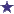# ratio, stock, stock solution

by Georgette
(Charleston, SC, USA)

How much of a 1:1000 stock is needed to make 360 ml of a 1:2400 solution?

### Comments for ratio, stock, stock solution

Average RatingRatingAnswer: 3600mL of original stock solution and 150mg of solute by: David Thank you for the question, Georgette!So we are starting with a 1:1000 stock solution, which is the same as = 0.1% StrengthWe want to end up with a 360mL of a 1:2400 stock solution.This is the same as a 0.0416% Strength.How do we get to the answer?We are going from a higher concentration (1:1000) to a lower concentration (1:2400).The question now is, how much sterile water are we going to add to make this to the correct concentration?In order to figure out how much diluent we will have at the end, we will multiply the volume (given, 360mL) by the percentage strength (given, 0.416%) which gives us 14.9999999, which we will round for simplicity's sake to 15mg. So now we have some known variables and one unknown variable:How much mixture (in mL) will we need at 0.1 percentage strength to equal 15mg in 360mL? 0.1% 15mg------ = ----------- X mL 360 mLCross multiply...360mL X 0.1% = X mL X 15mg360mL X 10 = 15mL X XmL3600 = 15mL X 240 mL150mg 15mg----- = ---------3600 360